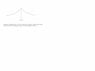# Derivative Question: Is There Another Explanation?

• Miike012
In summary, the conversation discusses the concept of continuity and the necessary condition for a graph to have a derivative at a point. The limit as delta(y)/delta(x) approaches zero is a key factor in determining if a graph is continuous at a certain point. However, it is important to note that this limit does not have to equal zero, it just needs to exist. The conversation also touches on the relationship between continuity and differentiability, and provides an example of a function that is continuous but not differentiable at a certain point. Additionally, the conversation clarifies the misconception of delta(y)/delta(x) approaching zero and explains the correct way to express it.

## Homework Statement

The chapter that I am reading is on continuity and talking about how inorder for a graph to have a derivative at some point say (x,y)... the limit as delta(y)/delta(x) must approach zero... If the limit approaches zero that means the graph is continuouse at point (x,y) therefore there is a derivative at that point... Please correct me if I am wrong...

Anyways my real question is in the attachemnt... can some one please help me understand... thank you.

Sorry the picture is small... the question is ... is there another explanation why there is no derivative at point (x,y) ...( in the picture) other than because the graph at that given point changes directions abruptly...?

#### Attachments

•aa.jpg
4.7 KB · Views: 432
Miike012 said:

## Homework Statement

... the limit as delta(y)/delta(x) must approach zero...

You sure you read that right? Consider the function given by the equation y=x; delta(y)/delta(x) approaches 1 as delta(x) approaches zero, and y=x is most certainly differentiable.

http://www.zweigmedia.com/RealWorld/calctopic1/contanddiffb.html

There is a derivative from the right as x approaches 1, and there is a derivative from the left as x approaches 1. These two derivatives are NOT equal. The point at x=1 is not differentiable.

So I am correct when I said that there is no derivative at that point?

And the reason why there is no derivative is because the left limit and the right limit... as the both approach the given point are not equal... is this correct?

Miike012 said:

## Homework Statement

The chapter that I am reading is on continuity and talking about how in order for a graph to have a derivative at some point say (x,y)... the limit as delta(y)/delta(x) must approach zero...
That limit, if it exists, is the derivative. The limit doesn't have to equal 0; it has to exist. So the important thing here is to know when a limit does, in fact, exist. (Or when does a limit not exist?)
If the limit approaches zero that means the graph is continuouse at point (x,y) therefore there is a derivative at that point... Please correct me if I am wrong...
We say f(x) is continuous at a point x=x0 if

$$\lim_{x \to x_0} f(x) = f(x_0)$$

Intuitively, this just means if you look at what f(x) is doing as you get close to x0, you see it's going to where it actually is at x0.

Continuity, however, doesn't imply differentiability. You can have a function which is continuous at a point, but not differentiable at that point. The other way, however, is true. If the function is differentiable at a point, it's also continuous there.

I added an attachment that says it approaches zero... Its copied straight from the book.

What there saying I think is
IF f(x) = x^2
x = 1 y = 1
x = 1.00001 y = 1.00002

Msec = (1 - 1.00002)/(1 - 1.00001) = 2
As you see... Delta(y) and Delta(x) both approach zero thus giving us the derivative at x = 1 Which is 2.

So yes... the average rate of change does approach zero.

#### Attachments

The derivative is calculated at a value of x say x0. When differentiating a function we expect delta y to approach zero because if delta y approaches some no-zero number or become infinite the quotient delta y/ delta x would not approach a definite number... that's what the picture says...

Miike012 said:
The derivative is calculated at a value of x say x0. When differentiating a function we expect delta y to approach zero because if delta y approaches some no-zero number or become infinite the quotient delta y/ delta x would not approach a definite number... that's what the picture says...

Yeah, that makes sense. Your problem was that you said that $\Delta y/\Delta x$ approaches zero. Although the two parts of the quotient do approach 0, the quotient itself doesn't.

Should I then say that Limit delta(y)/delta(x) as delta(x) approaches zero? Thats what they say in the book...